# General Relativity

### Flowchart of subjects leading up to studying GR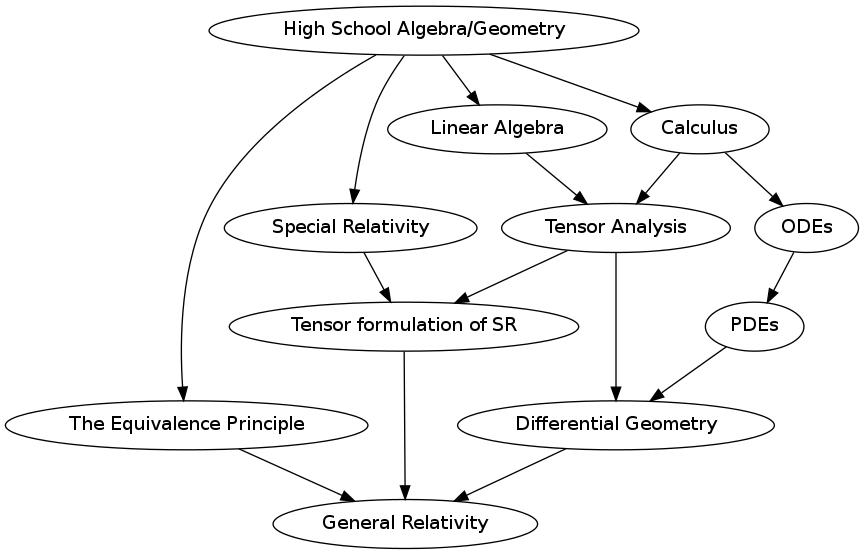### The Lay of the Land (from different perspectives)

• Outline of Topics
1. Overview
2. Special Relativity
3. The Equivalence Principle
4. Coordinate systems and coordinate transformations
5. Tensors and Invariant Equations
6. Tensor Math
7. Parallel Transport
8. The Tensor Derivative
9. The Schwarzschild solution
• The mathematical objects of interest
•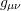– the Metric Tensor
•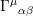– The Christoffel (Connection) Symbol
•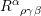– The Riemann (Curvature) Tensor
•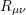– The Ricci Tensor
•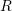– The Ricci Scalar
•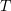– The Energy-Momentum Tensor
•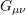– The Einstein Tensor
• The Equations
• Einstein’s Equation: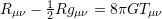• The Geodesic Equation: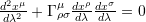• Coordinate Systems
• Boyer-Lindquist
• Eddington-Finkelstein
• Fermi normal
• Kerr-Schild
• Kruskal
• Painleve-Gullstrand
• Reimann normal
• Spacetimes
• de Sitter
• Friedmann-Robertson-Walker
• Kerr
• Kerr-Newman
• Majumdar-Papapetrou
• Minkowski
• Schwartschild
• Vaidya
• Horizons
• Apparent
• Cauchy
• Event
• Killing
• Trapping